# The Near Doubles Addition StrategyUse a known doubles fact and add or subtract from this.• The near doubles strategy uses the known doubles facts to assist with addition.
• Double one of the numbers in the addition and count on or back from this result.
• For example, 5 + 6 is one more than 5 + 5.
• Since 5 + 5 = 10, we know that 5 + 6 is one more than this.
• Therefore 5 + 6 = 11.
• To use the near doubles strategy, it helps to know the doubles facts below.• To calculate 9 + 8, we use the fact that 9 + 9 = 18.
• 9 + 8 must be one less than 9 + 9.
• Therefore 9 + 8 = 17.Supporting Lessons

#### The Near Doubles Strategy Video Lesson#### Near Doubles Activity

Click on the near doubles addition flashcards below to practise them:

+
+

=

# The Near Doubles Strategy

## What are Near Doubles?

Near doubles are maths facts that are close to doubles maths facts. For example, 3+4 is a near double because it is one more than 3+3. Near doubles are typically one or two larger or smaller than doubles facts.

Since we know that 3 + 3 = 6, we can work out 3 + 4 since it will be one larger.

3 + 4 = 7.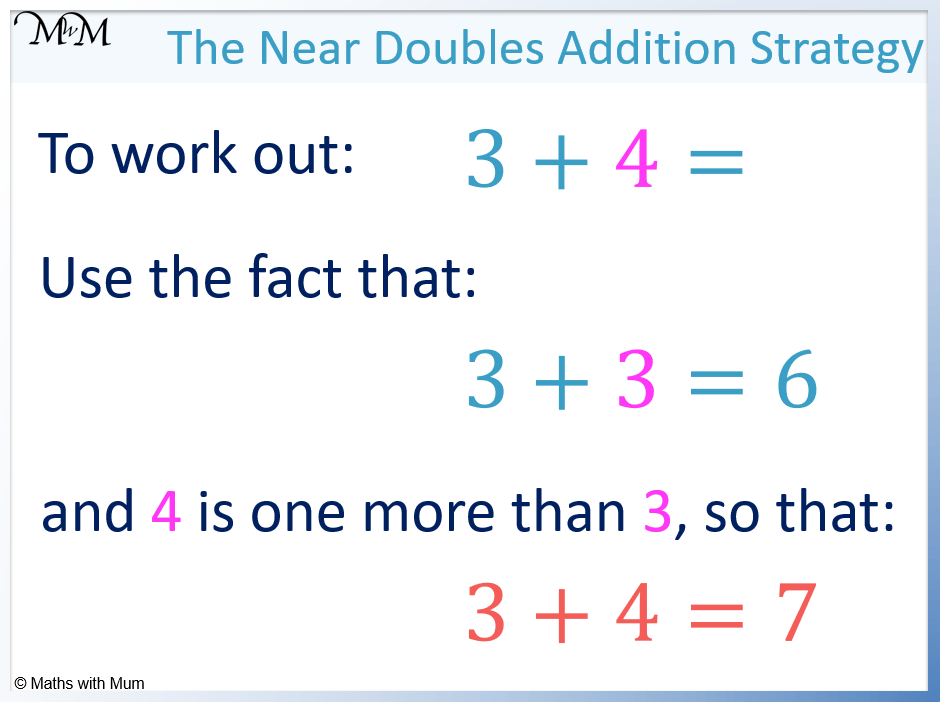## How to do Near Doubles Addition

To add numbers using the near doubles strategy, double one of the numbers in the addition. Then add or subtract the difference between the other number and the number that was doubled.

For example, add 6 + 7.

Doubling 6, we get 12.

Looking at the other number, 7 is one larger than 6 and so, we must add one more to our answer.

6 + 7 = 13.5 + 7 is a near double as it is close to 5 + 5.

Using the known double fact that 5 + 5 = 10, 5 + 7 must be two more than this and so, 5 + 7 = 12.Near doubles addition may be used when the numbers are below the doubles facts.

For example, 8 + 7 is close to the doubles fact 8 + 8 = 16. However, since 7 is one less than 8, we subtract one from the answer.

Therefore 8 + 7 = 15.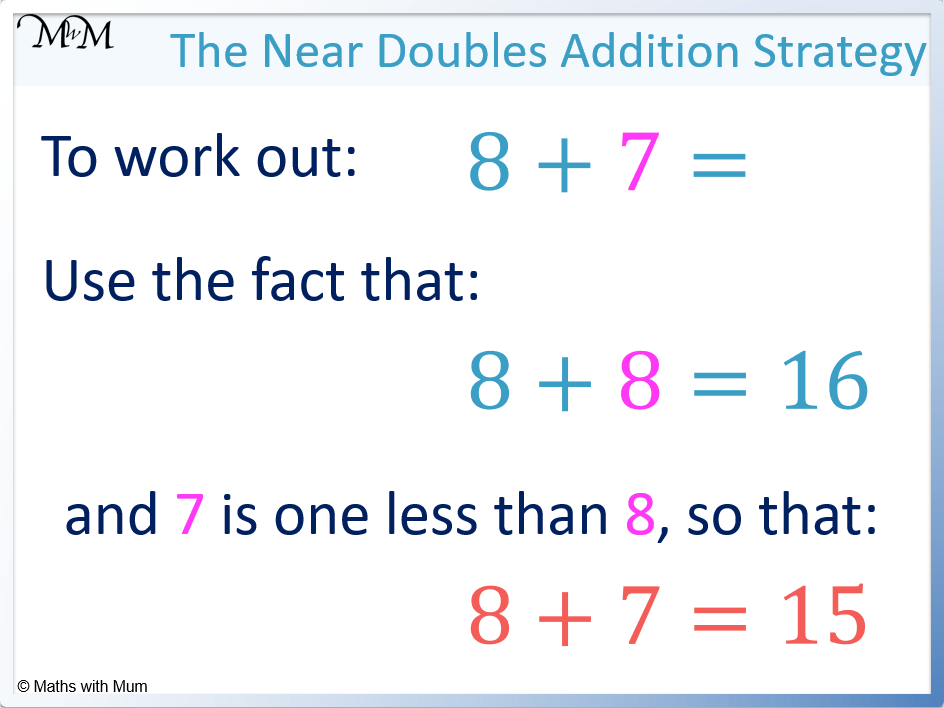## Teaching the Near Doubles Strategy

When teaching near doubles, first teach the doubles facts. Knowing these is necessary for the near doubles method to be effective. Using tens frames can be an effective teaching strategy for showing the comparative size of near doubles.

The near doubles addition strategy uses the knowledge of doubles facts as a crutch. This is because doubles facts are generally easily memorised.

The doubles facts that should be learnt are:

• 1 + 1 = 2
• 2 + 2 = 4
• 3 + 3 = 6
• 4 + 4 = 8
• 5 + 5 = 10
• 6 + 6 = 12
• 7 + 7 = 14
• 8 + 8 = 16
• 9 + 9 = 18
• 10 + 10 = 20Tens frames are useful for teaching the concept of a ‘near double’.

In the tens frame below, 3 + 3 is represented with pink counters.

It can be seen that 3 + 4 is shown with an extra green counter. The green counter is used to represent the difference needed.This can help conceptualise the idea of finding a difference to a known doubles fact.

It would be beneficial to first put the pink counters in place to represent the double. Then calculate the value of the double before adding the extra counters and then counting on from the previous total.

For example, 4 + 4 = 8 can be shown.

An extra counter can be placed next to one of the fours to make 4 + 5 = 9.

The idea is not to count the whole total again but simply count on from the known doubles fact of 4 + 4 = 8.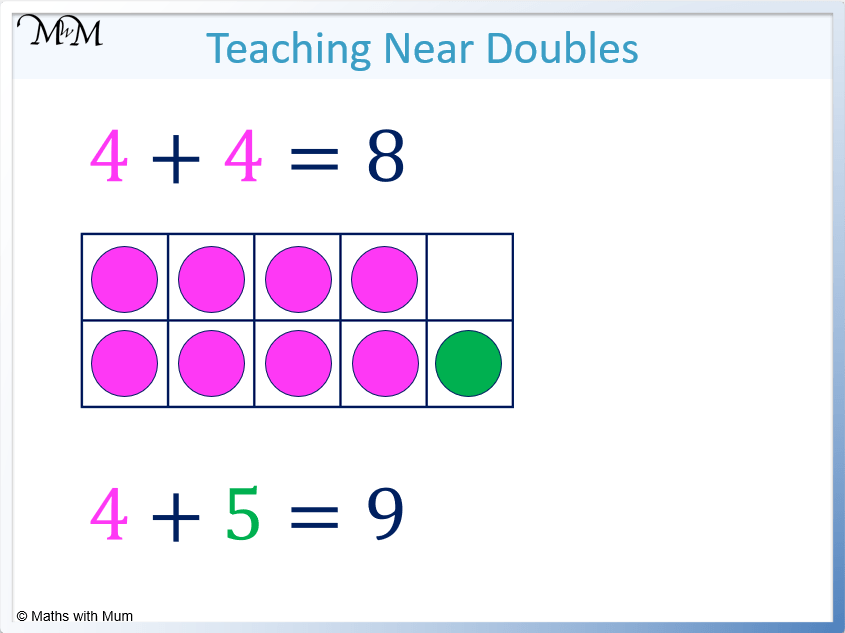Once the conceptual idea of a near double has been taught, it is beneficial to practise them using tasks that require recall such as flashcards.## Near Doubles Strategy for Subtraction

The near doubles strategy can be used for subtraction when the number being subtracted is close to half of the larger number. Use a known doubles fact and correct the result afterwards. For example, 12 – 6 = 6 and so 12 – 7 requires a further one to be subtracted. Therefore 12 – 7 = 5.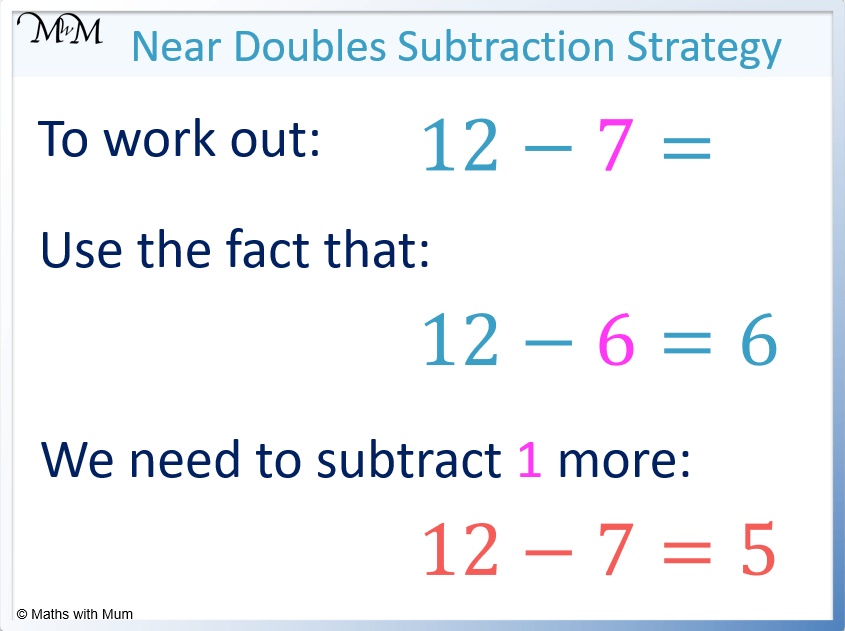For example, 15 – 8 can be calculated using the near doubles subtraction strategy.

We know that 16 – 8 = 8 because 8 doubled is 16.

15 – 8 must be one smaller than 16 – 8.

Therefore 15 – 8 = 7.Now try our lesson on Number Bonds to Ten where we learn the pairs of numbers that add to make 10.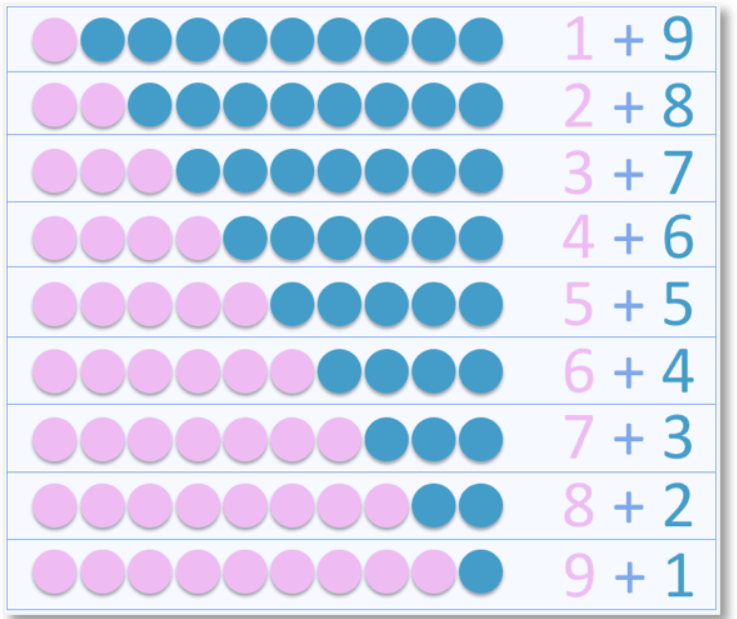error: Content is protected !!